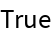#Function Repository Resource:

# ReferenceAngle

Compute the reference angle to a given input angle

Contributed by: Paco Jain (Wolfram Research)
 ResourceFunction["ReferenceAngle"][θ] gives the reference angle to the angle θ.

## Details and Options

ResourceFunction["ReferenceAngle"] takes all angles to be measured in radians.
The reference angle to a given input angle θ is defined geometrically as follows: First, start with a ray having its tail at the origin and pointing along the positive x axis. Next, rotate this ray counterclockwise about the origin by the angle θ. Finally, the reference angle to θ is the smallest positive angle that this ray makes with the x axis (either positive or negative).
The reference angle of θ in terms of θ can be found via the formula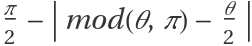.

## Examples

### Basic Examples

Compute a reference angle:

 In:=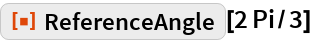Out=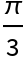Compute the reference angle for angles lying in each of the four quadrants:

 In:=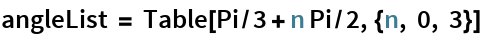Out=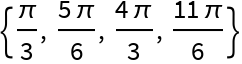In:=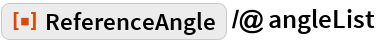Out=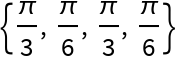Plot the reference angle function for all angles between 0 and 2π:

 In:=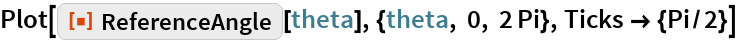Out=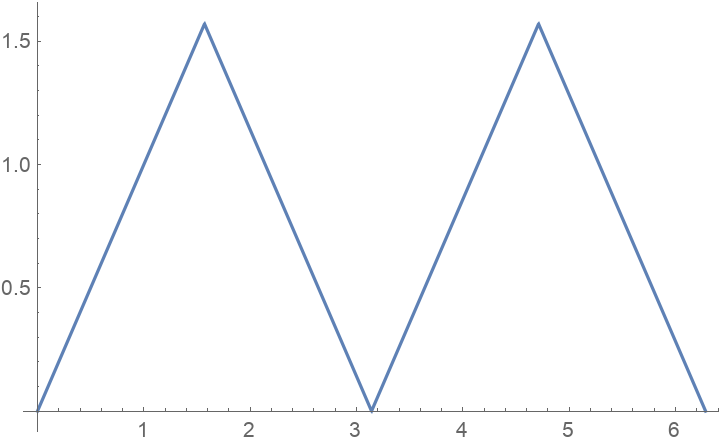### Scope

ReferenceAngle works directly on Quantity expressions with units of "Radians":

 In:=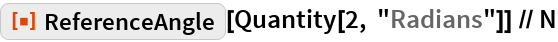Out=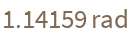### Properties and Relations

ReferenceAngle will remain unevaluated for non-numeric input:

 In:=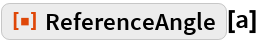Out=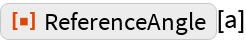ReferenceAngle throws away the sign of its input:

 In:=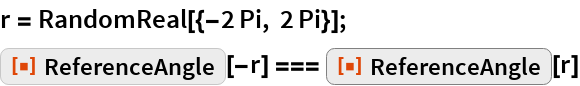Out=